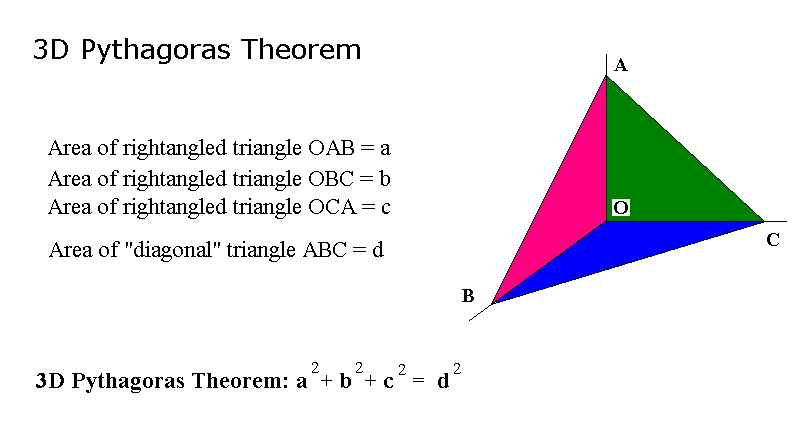Generalized Pythagoras Theorem

There are dozens of proofs for the Pythagoras theorem, here is the one I like best.Here is the three-dimensional version of the Pythagoras theorem.What is the proof? Is there an n-dimensional version of the Pythagoras theorem? There should be! Intrigued? Want to know the anwser? Well, we cannot just talk in terms of pictures, we need more sophisticated notations. Click here.

K. K. Nambiar